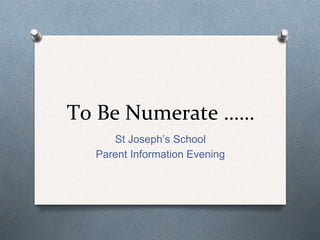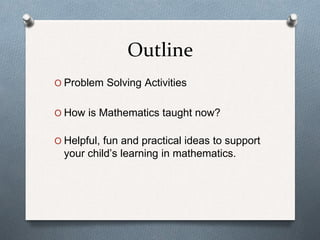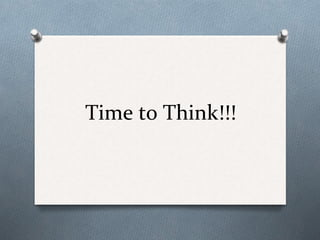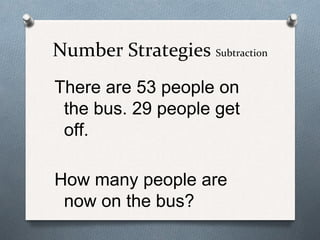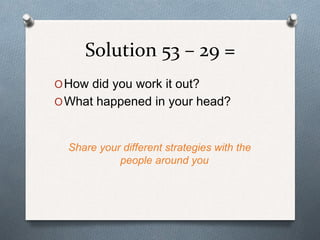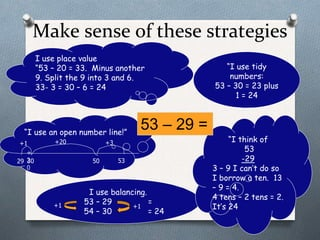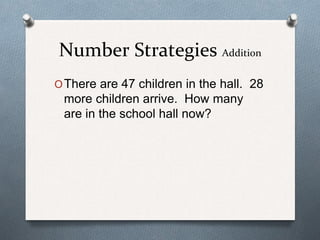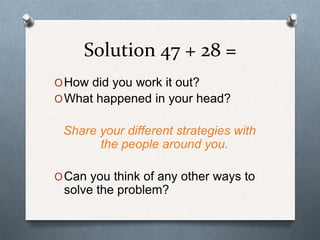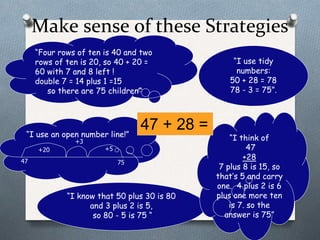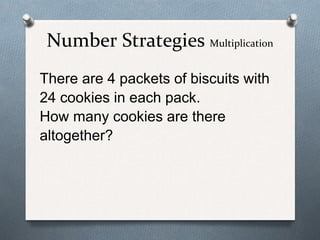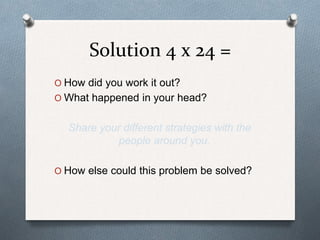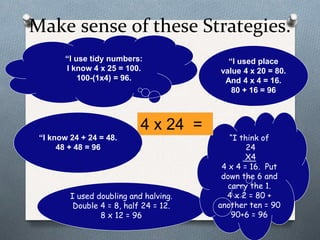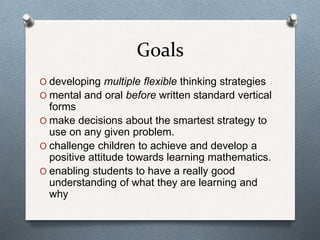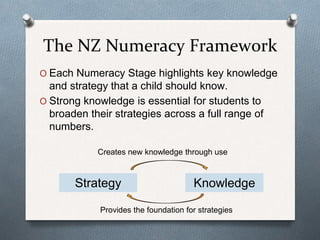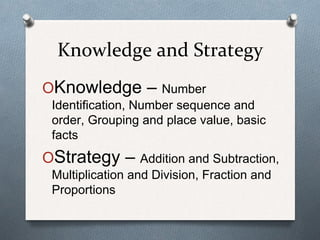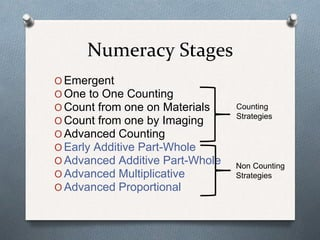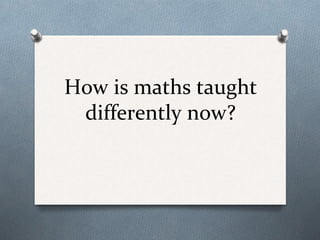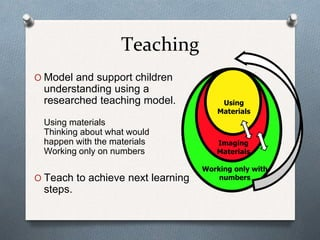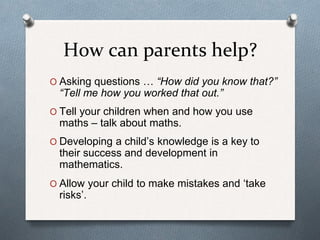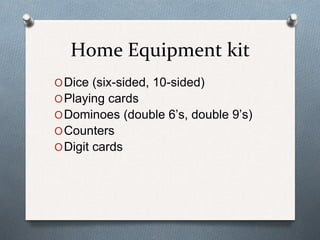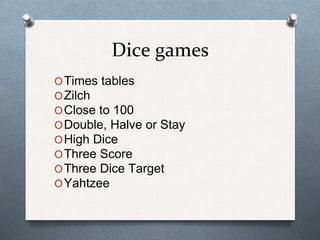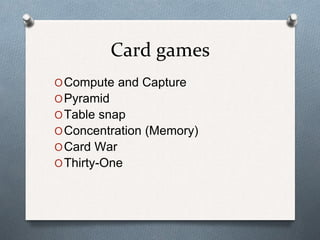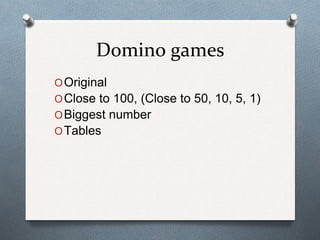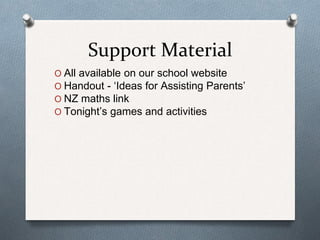1 of 25

### To be numerate St Joseph's School

1. To Be Numerate …… St Joseph’s School Parent Information Evening
2. Outline O Problem Solving Activities O How is Mathematics taught now? O Helpful, fun and practical ideas to support your child’s learning in mathematics.
3. Time to Think!!!
4. Number Strategies Subtraction There are 53 people on the bus. 29 people get off. How many people are now on the bus?
5. Solution 53 – 29 = OHow did you work it out? OWhat happened in your head? Share your different strategies with the people around you
6. Make sense of these strategies “I use tidy numbers: 53 – 30 = 23 plus 1 = 24 I use balancing. 53 – 29 = 54 – 30 = 24 “I think of 53 -29 3 – 9 I can’t do so I borrow a ten. 13 – 9 = 4. 4 tens – 2 tens = 2. It’s 24 “I use an open number line!” 53 – 29 = 29 53 +20 + 2 0 30 +3+1 50 +1 +1 I use place value “53 – 20 = 33. Minus another 9. Split the 9 into 3 and 6. 33- 3 = 30 – 6 = 24
7. Number Strategies Addition OThere are 47 children in the hall. 28 more children arrive. How many are in the school hall now?
8. Solution 47 + 28 = OHow did you work it out? OWhat happened in your head? Share your different strategies with the people around you. OCan you think of any other ways to solve the problem?
9. Make sense of these Strategies “Four rows of ten is 40 and two rows of ten is 20, so 40 + 20 = 60 with 7 and 8 left ! double 7 = 14 plus 1 =15 so there are 75 children” “I use tidy numbers: 50 + 28 = 78 78 - 3 = 75”. “I know that 50 plus 30 is 80 and 3 plus 2 is 5, so 80 - 5 is 75 “ “I think of 47 +28 7 plus 8 is 15, so that’s 5 and carry one. 4 plus 2 is 6 plus one more ten is 7. so the answer is 75” “I use an open number line!” 47 75 +3 +20 +5 47 + 28 =
10. Number Strategies Multiplication There are 4 packets of biscuits with 24 cookies in each pack. How many cookies are there altogether?
11. Solution 4 x 24 = O How did you work it out? O What happened in your head? Share your different strategies with the people around you. O How else could this problem be solved?
12. Make sense of these Strategies. “I used place value 4 x 20 = 80. And 4 x 4 = 16. 80 + 16 = 96 I used doubling and halving. Double 4 = 8, half 24 = 12. 8 x 12 = 96 “I think of 24 X4 4 x 4 = 16. Put down the 6 and carry the 1. 4 x 2 = 80 + another ten = 90 90+6 = 96 4 x 24 = “I use tidy numbers: I know 4 x 25 = 100. 100-(1x4) = 96. “I know 24 + 24 = 48. 48 + 48 = 96
13. Goals O developing multiple flexible thinking strategies O mental and oral before written standard vertical forms O make decisions about the smartest strategy to use on any given problem. O challenge children to achieve and develop a positive attitude towards learning mathematics. O enabling students to have a really good understanding of what they are learning and why
14. Developmental Stage Progression The New Zealand Number Framework
15. The NZ Numeracy Framework O Each Numeracy Stage highlights key knowledge and strategy that a child should know. O Strong knowledge is essential for students to broaden their strategies across a full range of numbers. Strategy Knowledge Creates new knowledge through use Provides the foundation for strategies
16. Knowledge and Strategy OKnowledge – Number Identification, Number sequence and order, Grouping and place value, basic facts OStrategy – Addition and Subtraction, Multiplication and Division, Fraction and Proportions
17. Numeracy Stages O Emergent O One to One Counting O Count from one on Materials O Count from one by Imaging O Advanced Counting O Early Additive Part-Whole O Advanced Additive Part-Whole O Advanced Multiplicative O Advanced Proportional Counting Strategies Non Counting Strategies
18. How is maths taught differently now?
19. Teaching O Model and support children understanding using a researched teaching model. Using materials Thinking about what would happen with the materials Working only on numbers O Teach to achieve next learning steps. Working only with numbers Imaging Materials Using Materials
20. How can parents help? O Asking questions … “How did you know that?” “Tell me how you worked that out.” O Tell your children when and how you use maths – talk about maths. O Developing a child’s knowledge is a key to their success and development in mathematics. O Allow your child to make mistakes and ‘take risks’.
21. Home Equipment kit ODice (six-sided, 10-sided) OPlaying cards ODominoes (double 6’s, double 9’s) OCounters ODigit cards
22. Dice games OTimes tables OZilch OClose to 100 ODouble, Halve or Stay OHigh Dice OThree Score OThree Dice Target OYahtzee
23. Card games OCompute and Capture OPyramid OTable snap OConcentration (Memory) OCard War OThirty-One
24. Domino games OOriginal OClose to 100, (Close to 50, 10, 5, 1) OBiggest number OTables
25. Support Material O All available on our school website O Handout - ‘Ideas for Assisting Parents’ O NZ maths link O Tonight’s games and activities